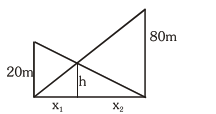# Two vertical poles of heights, 20m and 80m stand a part on a horizontal plane.`
Question:

Two vertical poles of heights, 20m and 80m stand a part on a horizontal plane. The height (in meters) of the point of intersection of the lines joining the top of each pole to the foot of the other, from this horizontal plane is :

1. 12

2. 15

3. 16

4. 18

Correct Option: , 3

Solution:by similar triangle

$\frac{h}{x_{1}}=\frac{80}{x_{1}+x_{2}}$      ......(1)

by $\frac{\mathrm{h}}{\mathrm{x}_{2}}=\frac{20}{\mathrm{x}_{1}+\mathrm{x}_{2}}$          .......(2)

by (1) and (2)

$\frac{x_{2}}{x_{1}}=4$ or $x_{2}=4 x_{1}$

$\Rightarrow \frac{\mathrm{h}}{\mathrm{x}_{1}}=\frac{80}{5 \mathrm{x}_{1}}$

or h = 16m